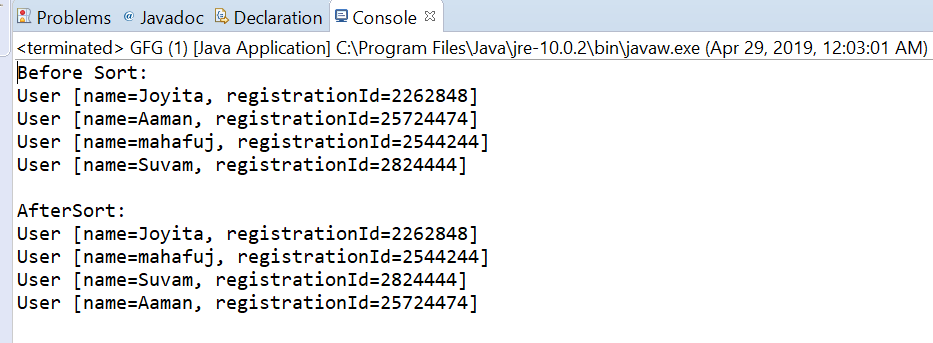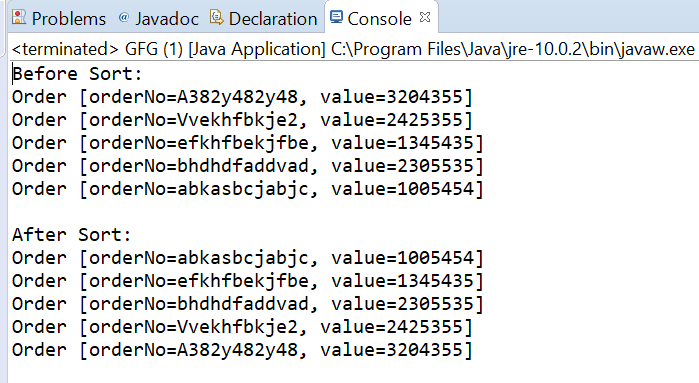# Comparator comparingLong() method in Java with examples

The comparingLong(java.util.function.ToLongFunction) method of Comparator Interface in Java accepts a function as parameter that extracts an long sort key from a type T, and returns a Comparator that compares by that sort key.The returned comparator is serializable if the specified function is also serializable.

Syntax:

```static <T> Comparator<T>
comparingLong(ToLongFunction <T> keyExtractor)
```

Parameters: This method accepts a single parameter keyExtractor which is the function used to extract the long sort key.

Return value: This method returns a comparator that compares by an extracted key

Exception: This method throws NullPolongerException if the argument is null.

Below programs illustrate comparingLong(java.util.function.ToLongFunction) method:
Program 1:

 `// Java program to demonstrate Comparator ` `// comparingLong(ToLongFunction)  method ` ` `  `import` `java.util.Arrays; ` `import` `java.util.Collections; ` `import` `java.util.Comparator; ` `import` `java.util.List; ` `public` `class` `GFG { ` `    ``public` `static` `void` `main(String[] args) ` `    ``{ ` ` `  `        ``// create some user objects ` `        ``User u1 = ``new` `User(``"Aaman"``, ``25724474``); ` `        ``User u2 = ``new` `User(``"Joyita"``, ``2262848``); ` `        ``User u3 = ``new` `User(``"Suvam"``, ``2824444``); ` `        ``User u4 = ``new` `User(``"mahafuj"``, ``2544244``); ` ` `  `        ``// before sort ` `        ``List list = Arrays.asList(u2, u1, u4, u3); ` `        ``System.out.println(``"Before Sort:"``); ` `        ``list.forEach(user -> System.out.println(user)); ` ` `  `        ``Collections.sort(list, ` `                         ``Comparator.comparingLong( ` `                             ``User::getregistrationId)); ` `        ``System.out.println(``"\nAfterSort:"``); ` `        ``list.forEach(user -> System.out.println(user)); ` `    ``} ` `} ` ` `  `class` `User ``implements` `Comparable { ` `    ``public` `String name; ` `    ``public` `long` `registrationId; ` ` `  `    ``public` `User(String name, ``long` `registrationId) ` `    ``{ ` `        ``this``.name = name; ` `        ``this``.registrationId = registrationId; ` `    ``} ` ` `  `    ``public` `int` `compareTo(User u1) ` `    ``{ ` `        ``return` `name.compareTo(u1.name); ` `    ``} ` ` `  `    ``public` `String getName() ` `    ``{ ` `        ``return` `name; ` `    ``} ` ` `  `    ``public` `void` `setName(String name) ` `    ``{ ` `        ``this``.name = name; ` `    ``} ` ` `  `    ``public` `long` `getregistrationId() ` `    ``{ ` `        ``return` `registrationId; ` `    ``} ` ` `  `    ``public` `void` `setregistrationId(``long` `registrationId) ` `    ``{ ` `        ``this``.registrationId = registrationId; ` `    ``} ` ` `  `    ``@Override` `    ``public` `String toString() ` `    ``{ ` `        ``return` `"User [name="` `+ name ` `            ``+ ``", registrationId="` `            ``+ registrationId + ``"]"``; ` `    ``} ` `} `

The output printed on console of IDE is shown below.
Output:Program 2:

 `// Java program to demonstrate Comparator ` `// comparingLong(ToLongFunction)  method ` ` `  `import` `java.util.Arrays; ` `import` `java.util.Collections; ` `import` `java.util.Comparator; ` `import` `java.util.List; ` `public` `class` `GFG { ` `    ``public` `static` `void` `main(String[] args) ` `    ``{ ` ` `  `        ``// before sort ` `        ``List list = Arrays.asList( ` `            ``new` `Order(``"A382y482y48"``, ``3204355``), ` `            ``new` `Order(``"Vvekhfbkje2"``, ``2425355``), ` `            ``new` `Order(``"efkhfbekjfbe"``, ``1345435``), ` `            ``new` `Order(``"bhdhdfaddvad"``, ``2305535``), ` `            ``new` `Order(``"abkasbcjabjc"``, ``1005454``)); ` `        ``System.out.println(``"Before Sort:"``); ` `        ``list.forEach(order -> System.out.println(order)); ` ` `  `        ``Collections.sort(list, ` `                         ``Comparator.comparingLong( ` `                             ``Order::getValue)); ` `        ``System.out.println(``"\nAfter Sort:"``); ` `        ``list.forEach(order -> System.out.println(order)); ` `    ``} ` `} ` ` `  `class` `Order ``implements` `Comparable { ` `    ``public` `String orderNo; ` `    ``public` `long` `value; ` ` `  `    ``public` `int` `compareTo(Order o1) ` `    ``{ ` `        ``return` `orderNo.compareTo(o1.orderNo); ` `    ``} ` ` `  `    ``public` `Order(String orderNo, ``long` `value) ` `    ``{ ` `        ``super``(); ` `        ``this``.orderNo = orderNo; ` `        ``this``.value = value; ` `    ``} ` ` `  `    ``@Override` `    ``public` `String toString() ` `    ``{ ` `        ``return` `"Order [orderNo="` `+ orderNo ` `            ``+ ``", value="` `+ value + ``"]"``; ` `    ``} ` ` `  `    ``public` `String getOrderNo() ` `    ``{ ` `        ``return` `orderNo; ` `    ``} ` ` `  `    ``public` `void` `setOrderNo(String orderNo) ` `    ``{ ` `        ``this``.orderNo = orderNo; ` `    ``} ` ` `  `    ``public` `long` `getValue() ` `    ``{ ` `        ``return` `value; ` `    ``} ` ` `  `    ``public` `void` `setValue(``long` `value) ` `    ``{ ` `        ``this``.value = value; ` `    ``} ` `} `

The output printed on console is shown below.
Output:Attention reader! Don’t stop learning now. Get hold of all the important Java and Collections concepts with the Fundamentals of Java and Java Collections Course at a student-friendly price and become industry ready.

My Personal Notes arrow_drop_upCheck out this Author's contributed articles.

If you like GeeksforGeeks and would like to contribute, you can also write an article using contribute.geeksforgeeks.org or mail your article to contribute@geeksforgeeks.org. See your article appearing on the GeeksforGeeks main page and help other Geeks.

Please Improve this article if you find anything incorrect by clicking on the "Improve Article" button below.

Article Tags :
Practice Tags :

Be the First to upvote.

Please write to us at contribute@geeksforgeeks.org to report any issue with the above content.